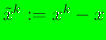## Prior weight function module

In priorweightfunction.f90, all subroutines and functions are contained within the priorweightfunction module. The radius of support for the nodal weight functions, metric D, and the prior are set in subroutine setrmaxandpriorweight(). This is done via a call to this function from an external program or from subroutine drivermaxent(), which is contained in the maxent module. The main program, main.f90, serves as an illustrative reference. The variables rmax, D, and prior are private variables, and hence once they are set, all functions within the module can access them. The weight functions are defined in function weightfunction(,,,). Here,runs fromto,, andrenders the nodal weight function support to be anisotropic. The variable, whereis the radius of support of the nodal weight function. The following weight functions are available:
1. uniform: a constant weight function, i.e.,.
2. cubic: the weight function is acubic spline function, which is given by(19)

3. quartic: the weight function is aquartic polynomial function, which is given by(20)

4. gaussian: the weight function is aGaussian function, which is given by(21)

5. gaussian-rbf: aGaussian radial basis function,, whereis a constant for each node.
If a different prior is desired, it can be added in function weightfunction(). The gradient is defined in function dweightfunction(), and the Hessian of the weight function is defined in function ddweightfunction().

N. Sukumar